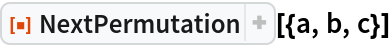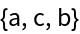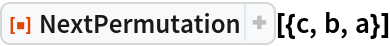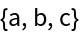#Function Repository Resource:

# NextPermutation

Get the next permutation in lexicographic order

Contributed by: Sriram V. Pemmaraju, Steven S. Skiena and Wolfram Staff
 ResourceFunction["NextPermutation"][p] gives the permutation following p in lexicographic order.

## Details and Options

The relative order of pairs of elements is as determined by the function Order.
The indexing of permutations relative to one another is as determined by the resource function PermutationIndex.
Permutations must be free of duplicates, or they may give unexpected results.

## Examples

### Basic Examples (1)

Get the next permutation in lexicographic order:

 In:=Out=### Properties and Relations (1)

The permutation that follows the highest-indexed one is the lowest-indexed one, wherein the elements are sorted:

 In:=Out=